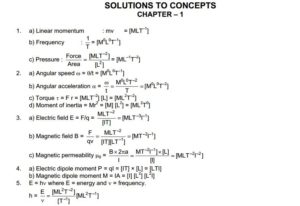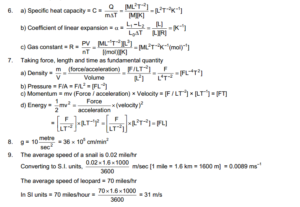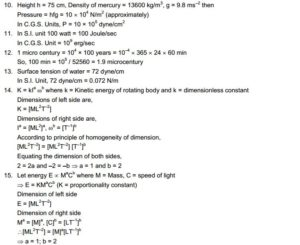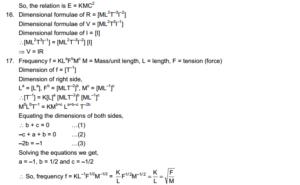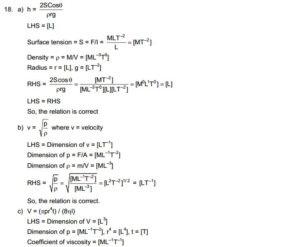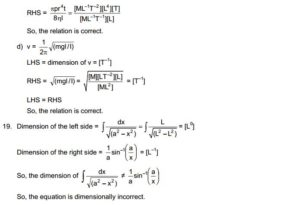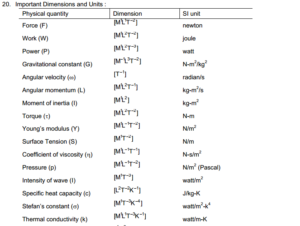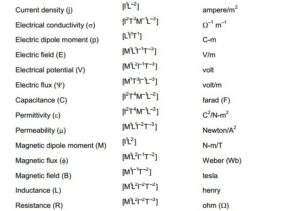Study Material

# HC Verma : Concept Of Physics Chapter 1 : Introduction To Physics SolutionWritten by

HC Verma Concept Of Physics Chapter 1 Solution is available here. You can also download it in pdf format. There are lots of consequences we have faced to publish this solution so please share it in social media.

Name of chapter 1 is Introduction to physics which contains some basic idea about applied physics. So Introduction to physics all the problem solution is covered in below given images.

### Introduction to Physics : Chapter 1 : Solution By HC Verma

The book starts with an chapter named Introduction to Physics and goes on to elucidate the concept of Mathematics and Physics in the second chapter. The author slightly talks about Kinematics in the third chapter. Then HC Varma draws the students attention toward concept of Forces. So download HC Verma Solution PDF From HERE.

• Download All Chapter Solution of HC Verma Part 1 & 2 Concept Of Physics